### IMO Shortlist 1985 problem 3

Kvaliteta:
Avg: 0,0
Težina:
Avg: 0,0
For any polynomial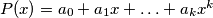$P(x)=a_0+a_1x+\ldots+a_kx^k$ with integer coefficients, the number of odd coefficients is denoted by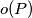$o(P)$. For$i-0,1,2,\ldots$ let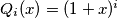$Q_i(x)=(1+x)^i$. Prove that if$i_1,i_2,\ldots,i_n$ are integers satisfying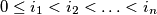$0\le i_1, then: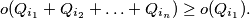Izvor: Međunarodna matematička olimpijada, shortlist 1985